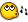# Filefindfirstfile

## Recommended Posts

Is there a way for it to search within subfolders of a folder?

Thanks

red

##### Share on other sites

Check the attributes of all returned files (FileGetAttirbute I think). If it has "D", then it's a directory, so start another FFFF/N loop.

##### Share on other sites
• Developers

Looking for a filename/mask in all subdirs?

You could do it this way with autoit syntax only:

```Dim \$FILES
Dim \$DIR="c:\winutil\autoit3\programs\test"; specify the directory to search

For \$X = 0 To UBound(\$FILES)-1
MsgBox(0,'test:' & \$X,\$FILES[\$X])
Next
Exit

Dim \$N_DIRNAMES; max number of directories that can be scanned
Local \$N_DIRCOUNT = 0
Local \$N_FILE
Local \$N_SEARCH
Local \$N_TFILE
Local \$N_OFILE
Local \$T_FILENAMES
Local \$T_FILECOUNT
Local \$T_DIRCOUNT = 1
; remove the end \ If specified
If StringRight(\$T_DIR,1) = "\" Then \$T_DIR = StringTrimRight(\$T_DIR,1)
\$N_DIRNAMES[\$T_DIRCOUNT] = \$T_DIR
; Exit if base dir doesn't exists
If Not FileExists(\$T_DIR) Then Return 0
; keep on looping until all directories are scanned
While \$T_DIRCOUNT > \$N_DIRCOUNT
\$N_DIRCOUNT = \$N_DIRCOUNT + 1
; find all subdirs in this directory and save them in a array
\$N_SEARCH = FileFindFirstFile(\$N_DIRNAMES[\$N_DIRCOUNT] & "\*.*")
While 1
\$N_FILE = FileFindNextFile(\$N_SEARCH)
If @error Then ExitLoop
; skip these references
If \$N_FILE = "." Or \$N_FILE = ".." Then ContinueLoop
\$N_TFILE = \$N_DIRNAMES[\$N_DIRCOUNT] & "\" & \$N_FILE
; if Directory than add to the list of directories to be processed
If StringInStr(FileGetAttrib( \$N_TFILE ),"D") > 0 Then
\$T_DIRCOUNT = \$T_DIRCOUNT + 1
\$N_DIRNAMES[\$T_DIRCOUNT] = \$N_TFILE
EndIf
Wend
FileClose(\$N_SEARCH)
; find all Files that mtach the MASK
\$N_SEARCH = FileFindFirstFile(\$N_DIRNAMES[\$N_DIRCOUNT] & "\" & \$T_MASK )
If \$N_SEARCH = -1 Then ContinueLoop
While 1
\$N_FILE = FileFindNextFile(\$N_SEARCH)
If @error Then ExitLoop
; skip these references
If \$N_FILE = "." Or \$N_FILE = ".." Then ContinueLoop
\$N_TFILE = \$N_DIRNAMES[\$N_DIRCOUNT] & "\" & \$N_FILE
; if Directory than add to the list of directories to be processed
If StringInStr(FileGetAttrib( \$N_TFILE ),"D") = 0 Then
\$T_FILENAMES  = \$T_FILENAMES & \$N_TFILE & @CR
\$T_FILECOUNT = \$T_FILECOUNT + 1
;MsgBox(0,'filecount ' & \$T_FILECOUNT ,\$N_TFILE)
EndIf
Wend
FileClose(\$N_SEARCH)
Wend
\$T_FILENAMES  = StringTrimRight(\$T_FILENAMES,1)
\$N_OFILE = StringSplit(\$T_FILENAMES,@CR)
Return( \$N_OFILE )
EndFunc  ;==>_GetFileList```
Edited by JdeB

Live for the present,
Dream of the future,
Learn from the past.##### Share on other sites

There are two other solutions here: http://www.hiddensoft.com/forum/index.php?...findpost&p=8487

.. I like letting DOS do all the recursion work, me## Create an account

Register a new account

• ### Recently Browsing   0 members

×

• Wiki

• Back

• #### Beta

• Git
• FAQ
• Our Picks
×
• Create New...